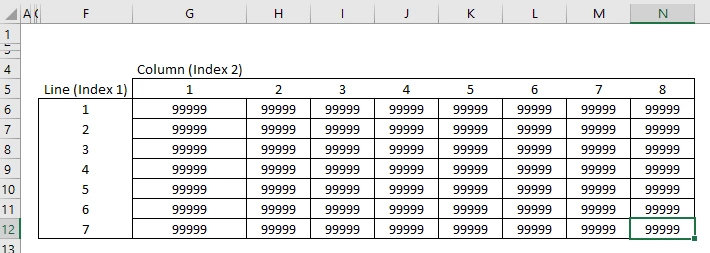Solved

# Dimension of Parameter in procedure

• Enthusiast
• 9 replies
Hi! I would like to ask what this error means and how I can solve this:

ExcelRetrieveParameter(FINAL DATA.xlsx, nC(v,T,w), C3:ALN22, , 0) failed: The dimension of parameter "nC(v,T,w)" is 3. It must be equal to or smaller than 2.

I hope anyone can help me with this. Thank you!
icon

Best answer by mateusarakawa 23 May 2019, 20:15

Hi, @pamjsnn.

Reading the Aimms function reference, the second argument of this excel function states that the parameter dimension can be 0, 1 or 2. In your case, parameter "nC" has 3 indexes {v,T,w}.

Thus, Spreadsheet::RetrieveParameter could only read a table with two indexes max., as shown below (Range "G6:N12"):View original

### 2 replies

Hi, @pamjsnn.

Reading the Aimms function reference, the second argument of this excel function states that the parameter dimension can be 0, 1 or 2. In your case, parameter "nC" has 3 indexes {v,T,w}.

Thus, Spreadsheet::RetrieveParameter could only read a table with two indexes max., as shown below (Range "G6:N12"):